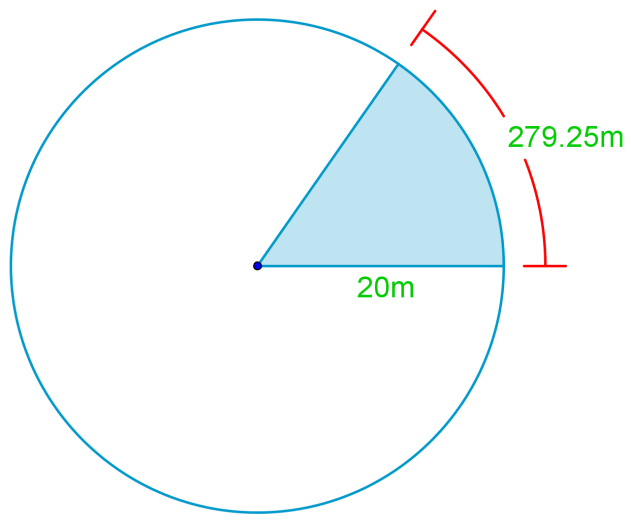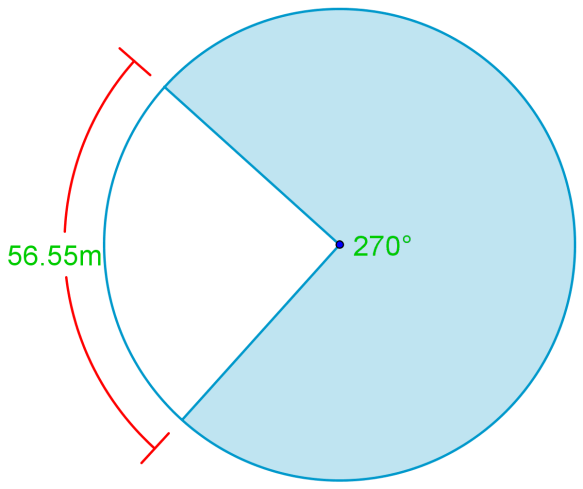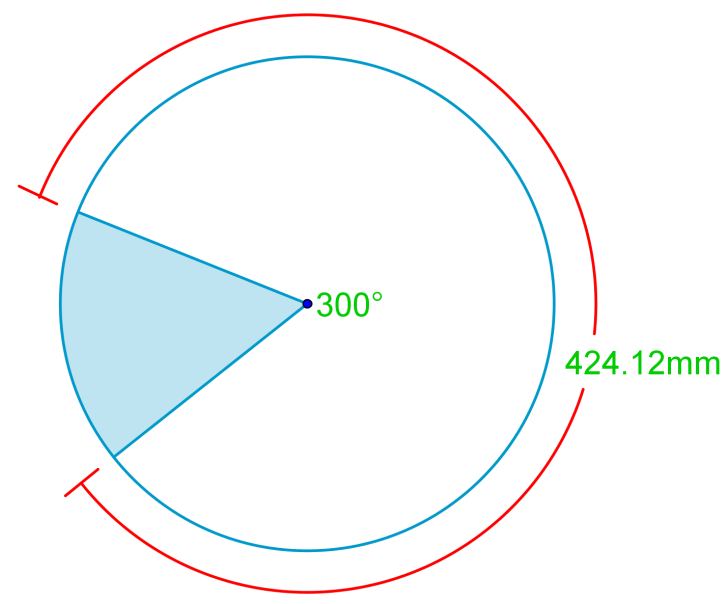# Areas and sectors of circles#### All You Need in One Place

Everything you need for better marks in primary, GCSE, and A-level classes.#### Learn with Confidence

We’ve mastered the UK’s national curriculum so you can study with confidence.#### Instant and Unlimited Help

0/1
##### Intros
###### Lessons
1. How to find the area of a sector?
0/8
##### Examples
###### Lessons
1. Find the areas of the circles with the given information below.
2. Diameter is 18 mm
2. Find.
i) Area of the circle
ii) The area of the shaded sector

1.2.3.3. Linda wants to get pizzas for her house party. She has invited 10 friends over. A 12-inch pizza is enough for 4 people. An 8-inch pizza is $7, while a 12-inch pizza is$12. How many 8-inch pizzas and 12-inch pizzas does she need to order, if:
1. she wants to spend the least amount of money?
2. she wants to have 4 pizzas and spend as little as possible?
3. she wants to eat as much outer crust as possible?

## How to find the area of a circle

To begin this lesson on finding the area of a sector, we'll first have to start with the area of a circle. A circle with a radius r has an area A found through:

$A=\pi r^2$

Do you know how to then find the circumference? The circumference C can be found with the formula of:

$C=2 \pi r$

As you can see, in both cases, for both the area and the circumference, we're dealing with the whole circle. Let's take a look at if we were just dealing with a section of the circle.

We can divide a circle into sectors. You can imagine sectors as a radius that extends from the circle's center that then goes around the circumference for a bit, then turns to go down a radius once again to meet at the center. You'll get a sector of a circle that looks like a slice of pie. It has an angle at the center of the slice (or the tip of the slice, if you prefer) that is called the "central" angle.

An important term we're going to have to learn is arc length. The arc length of a sector is the portion of circumference that the sector takes up from the whole circle. It is the portion of the circumference subtended by the central angle.

Keeping in mind the circle's total area, the circumference, and the arc length, we can now learn how to find the area of a section of a circle.

## How to find the area of a sector

Through an example question, we'll demonstrate how the relationship between the area of a section and total area can help you find the section's area. There is an area of a sector formula that can help you out which we'll share below, so don't worry about it for now.

Question:

Find the

(i) Area of the circle

(ii) The area of the shaded sector

Solution:

(i) To find area of a circle, use the formula $\pi r^2$

So the area is $\pi r(20)^2=1256.6m$

We simply need to substitute in the radius of 20m to find the answer.

(ii)

$\frac{area\;of\;section}{total\;area} = \frac{arc\;length}{circumference}$

$\frac{area\;of\;section}{1256.6} = \frac{279.25}{2 \pi (20)}$

$area\;of\;section = \frac{279.25(1256.6)}{2 \pi (20)}$

$area\;of\;section = 2792\;m^2$

What exactly are we doing here? In this question, we're finally trying to find the area of a sector. Since the sector is a portion of the total circle, there's a relationship between them. Likewise, there's a relationship between the section of the circumference that's a part of the sector (the arc length), versus the whole circumference. Their ratios are the same! Or rather, their proportions are the same.

That's why we use the formula of:

$\frac{area\;of\;section}{total\;area} = \frac{arc\;length}{circumference}$

This helps us use the proportions to work out the unknown. In this case, the unknown is the area of the sector. We're given the arc length (279.25m), we've got the circumference from the formula of $C = 2 \pi r$, and lastly, we've calculated the total area from part (i) of the question. The rest of the question is just a matter of substituting in these numbers to the formula and then isolating the area of a sector (the unknown) onto one side to find the answer.

This online interactive sector area of a circle can be dragged so you can explore how the area sector changes as its central angle changes. It's worth checking out to solidify your understanding of the relationships explored in this lesson for circles.